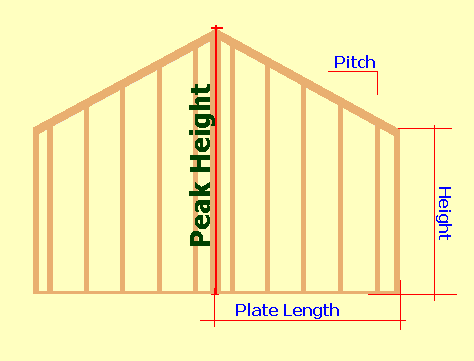Spike's Calculators

# Gable (Rake) Wall Framing - Wall Height

Calculate the height of a gable (rake) wall based on roof slope and half of the wall's horizontal length the "rake run".

##### Example calculation:
bottom plate length = 12' - 6"
roof slope = 5 : 12

convert plate length amount of 12' - 6" into inches
12 * 12 + 6 = 150 inches

roof slope; divide the rise by the run
5 ÷ 12 = 0.4166667

multiply the length of the plate by this amount
150 * 0.4166667 = 62.5

The 62.5 inches is the increase in height for the rake wall from its lowest to the highest point.

If your wall has a starting height of 96" (8 feet) the rake wall will have a total height of 96 + 62.5 = 158.5 inches = 13' - 2 1/2"Calculates the "peak height" of a gable wall!!

### Rake Wall Height

Slope Ratio :12
Plate Length ft in n
d
Lower Wall Height ft in n
d

#### Results:

 Decimal Inch in Inches in Feet and Inches ft in

#### Calculation

1. roof slope ratio of inches per foot
2. length of bottom plate in feet and inches (rake run)
3. lower wall height

#### Results

1. peak height in decimal inches
2. peak height in inches
3. peak height in feet and inches